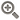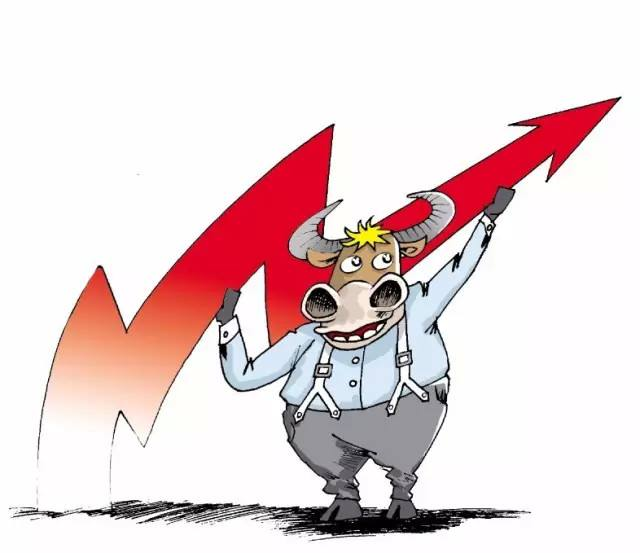﻿ ​盈利能力成长性与内在价值系列谈-点掌财经• 综合
• 股票
• 板块
• 嘉宾
• 课程
• 基金
• 经理
• 说说
• 掌评
• 消息
• 好看
• 话题

# ​盈利能力成长性与内在价值系列谈1，品牌效应使其拥有定价权，35年间产品销售价格年均上涨了5.53%，销售收入的增长主要来源于价格的上涨，而不是生产规模的扩张，因此，资本性支出很低；

2，产品销售以现款结算，没有应收账款；

3，生产和分销周期很短，存货周转快，存货占用资金低。

P1=0.3/(10%-2%)=3.75(元)

P2=0.5/(10%-0)=5(元)

PB=1+(ROE-R)/(R-g)(1)

R=D1/P+g(2)

R=D1/P+g

=D1/P+(E-D1)/B

=E/B+D1(1/P-1/B)

=ROE+(1-P/B)×D1/P(3)

#### 最新发布• 手机号码：

请输入手机号码，您的个人信息严格保密，请放心

• 图形验证码：

请输入右侧图形验证码

• 短信验证码：

请输入接收的短信验证码

获取短信验证码
X• 10牛
• 30牛
• 50牛
• 其它